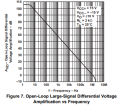# Need help understanding Gain of Op amp

#### Psf1575

Joined Mar 2, 2018
16
hey everyone, im confused on the gain quantities of the 741 op amp. Im so confused im having trouble figuring out how to even ask this.

I know the open loop gain is 100,000. But theres also different gains at different frequencies after the cutoff frequency on a bode plot. So in a plot, you would draw the midband gain as 100,000 then when you hit the bandwith point, it decreases until you hit the unity gain frequency. Whats the difference between these gains?

When solving problems, the closed loop gain I calculate via formula for inverting and non inverting configurations is the midband gain in a bode plot, then we do the same thing as with the open loop op amp 741.

Why is the gain we calculate always the midband gain when we have all these different gains at different frequencies?

#### ericgibbs

Joined Jan 29, 2010
15,484
hi P.
Look at Fig '7 of this PDF.
Ask if you need further info.
E

#### Attachments

• 1.1 MB Views: 19

#### ericgibbs

Joined Jan 29, 2010
15,484
Why is the gain we calculate always the midband gain when we have all these different gains at different frequencies?
hi,
You have this the wrong around.
When the gain is set by Rin and Rfb, this sets the maximum frequency possible at that gain, as shown on the d/s gain plot fig 7, in this example.
E

#### crutschow

Joined Mar 14, 2008
29,752
Below is the Figure 7 that Eric referenced.
Note that the maximum open-loop gain occurs only at frequencies below about 4Hz and then drops off with frequency at a rate of 20dB per decade.
The frequency response for a particular closed-loop gain is where the gain intersects this graph line.
Thus for a closed-loop gain of 40dB, the -3dB frequency response corner would be about 8kHz.#### Psf1575

Joined Mar 2, 2018
16
hi,
You have this the wrong around.
When the gain is set by Rin and Rfb, this sets the maximum frequency possible at that gain, as shown on the d/s gain plot fig 7, in this example.
E
I see,
hi,
You have this the wrong around.
When the gain is set by Rin and Rfb, this sets the maximum frequency possible at that gain, as shown on the d/s gain plot fig 7, in this example.
E
thanks for the reply! So, if we have an inverting op amp, whose gain is -Rf/R1, that gain will be the maximum gain of the system until we get to the cutoff frequency where it starts to decrease right?

#### Psf1575

Joined Mar 2, 2018
16
Below is the Figure 7 that Eric referenced.
Note that the maximum open-loop gain occurs only at frequencies below about 4Hz and then drops off with frequency at a rate of 20dB per decade.
The frequency response for a particular closed-loop gain is where the gain intersects this graph line.
Thus for a closed-loop gain of 40dB, the -3dB frequency response corner would be about 8kHz.

View attachment 160999
So the open loop voltage gain specified by the data sheet is going to be the maximum gain the op amp can have until it hits the cutoff frequency of 4 KHz. And for the closed loop the bandwith increased to 8 KHz since we reduced the gain using negative feedback?

Joined Mar 10, 2018
4,057
Keep in mind for a one pole compensated OpAmp (a somewhat idealized situation
that ignores external parasitics) the GBW is a constant.

So say you have an OpAmp whose Aol is 100K at 10 Hz, then its GBW = 1,000,000.
Then if you set Rs for a G = 10 then 10 x BW = GBW = 1,000,000 or BW = 100 Khz
for G = 10.

Many current day OpAmps are 2 pole, but the second pole is far enough out from
dominant pole that constant GBW still applies.

This above is not exact, but close enough.

http://www.analog.com/media/en/training-seminars/tutorials/MT-033.pdf

Regards, Dana.

Last edited:

#### atferrari

Joined Jan 6, 2004
4,593
I got used to think, for the calculation, that I am working with DC (f0 =0 Hz).